## Finding problems using unsupervised image categorization

#### Analysis

When an extended set of parameters is encountered, as in the example presented in this article, the most obvious means of reducing this feature space is to perform PCA (see the "PCA" sidebar). Listing 5 separates the data of each detector type to perform PCA on each group independently.

Listing 5

Independent DataFrames for Detector Types

```# Map filename Extension to Detector Type
dectorType = {
"Specular": "000",
"Phase": "001",
"Scatter": "002"
}
import pandas as pd
# Select the features for a single Detector Type
# Specular Detector
imgFeaturesSpecular = imgFeatures.filter( items =  wafStepIMGList_df[wafStepIMGList_df['EXT'] == dectorType["Specular"] ]['IMAGE_ID'] , axis=0)
# Phase Detector
imgFeaturesPhase = imgFeatures.filter( items =  wafStepIMGList_df[wafStepIMGList_df['EXT'] == dectorType["Phase"]]['IMAGE_ID'] , axis=0)
# Scatter Detector
imgFeaturesScatter = imgFeatures.filter( items =  wafStepIMGList_df[wafStepIMGList_df['EXT'] == dectorType["Scatter"]]['IMAGE_ID'] , axis=0)```

Standardizing the values within each component prevents bias resulting from individual components simply because they contain larger values (see Listing 6). This step is provided by scikit-learn and imported via sklearn .

Listing 6

Standardizing Values

```from sklearn.preprocessing import StandardScaler
stdimgFeaturesSpecular = pd.DataFrame(StandardScaler().fit_transform(imgFeaturesSpecular), index=imgFeaturesSpecular.index, columns=imgFeaturesSpecular.columns)
stdimgFeaturesPhase = pd.DataFrame(StandardScaler().fit_transform(imgFeaturesPhase), index=imgFeaturesPhase.index, columns=imgFeaturesPhase.columns)
stdimgFeaturesScatter = pd.DataFrame(StandardScaler().fit_transform(imgFeaturesScatter), index=imgFeaturesScatter.index, columns=imgFeaturesScatter.columns)```

Once the data is in a fit state to perform PCA, the transformation can be performed via another sklearn sub-library  (see Listing 7). The decomposition into two components allows you to plot the results conveniently.

Listing 7

Extracting PCA Components into Two Axes

```# import PCA from sklearn library
from sklearn.decomposition import PCA
pca = PCA(n_components=2)
#tranform the given data set using PCA
principalDfSpecular = pd.DataFrame(data =pca.fit_transform(stdimgFeaturesSpecular), index=stdimgFeaturesSpecular.index, columns = ['PC1', 'PC2'])
principalDfPhase = pd.DataFrame(data =pca.fit_transform(stdimgFeaturesPhase), index=stdimgFeaturesPhase.index,  columns = ['PC1', 'PC2'])
principalDfScatter = pd.DataFrame(data =pca.fit_transform(stdimgFeaturesScatter), index=stdimgFeaturesScatter.index, columns = ['PC1', 'PC2'])
#principalDf['PC1']```

In order to simplify subsequent data handling, a label column is added to each DataFrame to identify the detector type (specular, phase, or scatter), and all DataFrames are subsequently concatenated together (see Listing 8).

Listing 8

```principalDfSpecular['Detector'] = 'Specular'
principalDfPhase['Detector'] = 'Phase'
principalDfScatter['Detector'] = 'Scatter'
principalDf = pd.concat([principalDfSpecular, principalDfPhase, principalDfScatter])```

The `jointplot` functions available from the Seaborn library provide a succinct way to visualize any structure that may have been revealed by PCA (see Listing 9).

Listing 9

PCA Data for Each Detector Type

```import seaborn as sns
from matplotlib import pyplot as plt
plt.figure(figsize=(8,5))
sns.jointplot(data=principalDf[principalDf['Detector'] =='Scatter'], x='PC1', y='PC2', kind="hex", joint_kws=dict(bins='log'))```

The PCA density plots in Figure 2 show these results: Not only has the structure been revealed, but the structure is also distinctly different for each detector type.Figure 2: PCA density plots are shown here for the specular, phase, and scatter images.

To quantify this structure, some form of clustering needs to be applied to the PCA-transformed data. A natural choice is K-means clustering. However, in this case, the results are generally unsatisfactory at separating meaningful structures. For example in Figure 3, while the grey cluster and red random points are reasonably well separated, the other group of data points are crudely segmented, without regard for the internal structure. This is not necessarily always the case, and other examples exist where K-means clustering is an appropriate option .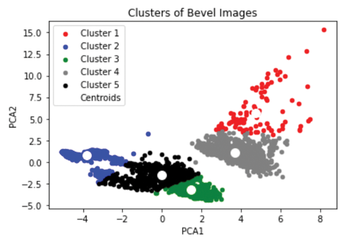Figure 3: K-means clustering has been applied to the PCA feature space.

Applying a DBSCAN clustering algorithm  produced much more promising results. Listing 10 applies this clustering algorithm with a minimum number of samples (22 in this example) per cluster to the two PCA components generated in Listing 7. The results of the DBSCAN clustering algorithm can be visualized with the help of the Matplotlib library, as shown in Listing 11. Figure 4 shows the results after the PCA data has been clustered using DBSCAN.

Listing 10

Applying the DBSCAN Algorithm

```from sklearn.cluster import DBSCAN
from sklearn import metrics
# Extract PCA vales into an array to perform clustering
X = df.iloc[:, [0, 1]].values
type(X)
# Compute DBSCAN
db = DBSCAN(eps=0.35, min_samples=22).fit(X) # DEFAULT
labels = db.labels_
# Number of clusters in labels, ignoring noise if present.
n_clusters_ = len(set(labels)) - (1 if -1 in labels else 0)
n_noise_ = list(labels).count(-1)
print("Number of clusters: %d" % n_clusters_)
print("Number of random points: %d" % n_noise_)
print("Silhouette Coefficient: %0.3f" % metrics.silhouette_score(X, labels))```

Listing 11

Plotting DBSCAN Clustering

```import matplotlib.pyplot as plt
unique_labels = set(labels)
colors = [plt.cm.Spectral(each) for each in np.linspace(0, 1, len(unique_labels))]
for k, col in zip(unique_labels, colors):
if k == -1:
col = [0, 0, 0, 1]
plt.plot(xy[:, 0],xy[:, 1],"o",markerfacecolor=tuple(col),markeredgecolor="k",markersize=6 )
plt.plot(xy[:, 0],xy[:, 1],"o",markerfacecolor=tuple(col),markeredgecolor="k",markersize=3)
plt.title("Estimated number of clusters: %d" % n_clusters_)
plt.show()```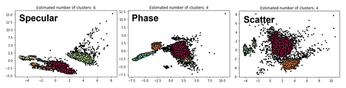Figure 4: The PCA parameter data is clustered using DBSCAN for specular, phase, and scatter images.

To visualize the differences between bevel images that were assigned different cluster IDs, the IDs must be merged with the DataFrame containing the images' and wafers' metadata from which the IDs were obtained (see Listing 12).

Listing 12

Merging Cluster IDs

```Xlabels = pd.DataFrame(np.append(X, labels.reshape((4281, 1)), axis=1))
Xlabels.columns = ['PC1', 'PC2', 'Cluster']
# Copy IMAGE_ID from the index to a column
df['IND'] = df.index
# Merge Cluster IDs with the IMAGE_IDs, using the PCA values
df = pd.merge(df, Xlabels, on=["PC1", "PC2"])
# Reset Index
df.set_index('IND', drop=True, inplace=True)
df.index.name = None
# Merge the Clustered PCA dataframe with the Image and Wafer metadata
df['IMAGE_ID'] = df.index
dfPLUS = df.merge(wafStepIMGList_df, on='IMAGE_ID')
dfPLUS = dfPLUS.merge(wafStepList_df, on=('WAF_ID','STEP_ID'))
dfPLUS['LOT_GRP'] = dfPLUS['WAF'].str.replace('[^A-Z]+', '')
# Pickle individual DataFrame with Clustered data to the filesystem
#dfPLUS.to_pickle('clusteredSpecularImgPLUS.pkl')
#dfPLUS.to_pickle('clusteredPhaseImgPLUS.pkl')
dfPLUS.to_pickle('clusteredScatterImgPLUS.pkl')```

I suspected much of the PCA chart structure was driven by wafer product differences. Listing 13 uses the Seaborn and Matplotlib libraries to generate a faceted chart to illustrate these differences (see Figure 5). Figure 5 shows a clear product type dependence, which can be seen in the overlap in the parameter space between the product types identified by A, B, and C, as well as those identified by X, Y, and Z.

Listing 13

Faceted Plot of Cluster IDs by Product Type

```import seaborn as sns
import matplotlib.pyplot as plt
sns.set(style="ticks", color_codes=True)
g = sns.FacetGrid(dfPLUS, col="PROD", col_wrap=4,  hue="Cluster")
g = g.map(plt.scatter, "PC1", "PC2", edgecolor="w")
plt.show()```Figure 5: The faceted chart generated from the PCA feature space shows the product type dependence.

#### Image Display

Because I did not start with a classification, I have nothing to compare the cluster IDs with, so I cannot construct a confusion matrix or calculate the precision or recall for this method. I can, however, visually compare the images within different cluster IDs for specific product types. To achieve this, you must identify the images within each category (the distinct clusters and the unclustered images) with the greatest distance from the center of the clusters. This first requires that the centroids be identified for each product type and detector type. Then the distance is calculated from the appropriate centroid to each individual datapoint (see Listing 14).

Listing 14

Product-Detector Cluster Centers

```import numpy as np
dfPLUS['PROD_Detect'] = dfPLUS['PROD'] + '_' + dfPLUS['Detector']
clusterCenters = dfPLUS[dfPLUS['Cluster'] > -1][['PROD_Detect', 'Cluster','PC1','PC2']].groupby(['PROD_Detect']).mean()
clusterCenters.index.name = None
dfPLUS['Delta1'] = \
dfPLUS.apply(lambda x: np.round_(x.PC1-clusterCenters.loc[x.PROD_Detect].PC1, 6), axis=1)
dfPLUS['Delta2'] = \
dfPLUS.apply(lambda x: np.round_(x.PC2-clusterCenters.loc[x.PROD_Detect].PC2, 6), axis=1)
dfPLUS['Delta'] = np.sqrt(dfPLUS['Delta1']**2 + dfPLUS['Delta2']**2)```

Once the distances from the centers are known, the most distant images can be identified for each product and detector type using the `findExtremeImgs` function in Listing 15. This function returns two DataFrames: One for the abnormal or unclustered images and one for the normal or clustered images. The number of images within each category is specified by the `mcols` value, which is set to a value of `5` in my example.

Listing 15

DataFrames for Normal and Abnormal Images

```def findExtremeImgs (detectorType, prodName):
mcols = 5
abnormalImg = dfPLUS[(dfPLUS['Detector'] == detectorType) & (dfPLUS['PROD'] == prodName) & (dfPLUS['Cluster'] < 0)]\
[['PROD_Detect', 'Cluster','PC1','PC2','Delta','FILENAME']].\
sort_values(['Delta'], ascending=False).\
groupby(['PROD_Detect','Cluster']).\
normalImg = dfPLUS[(dfPLUS['Detector'] == detectorType) & (dfPLUS['PROD'] == prodName) & (dfPLUS['Cluster'] >= 0)]\
[['PROD_Detect', 'Cluster','PC1','PC2','Delta','FILENAME']].\
sort_values(['Delta'], ascending=False).\
groupby(['PROD_Detect','Cluster']).\
return abnormalImg, normalImg```

The `displayExtremeImgs` function in Listing 16 will accept the lists of extreme images and display them in a grid format.

Listing 16

Grid Display of Normal and Abnormal Images

```def displayExtremeImgs (detectorType, thisProd, abnormalImg, normalImg):
thisCols = abnormalImg['Cluster'].size
clusterIDlist = pd.DataFrame(np.concatenate((np.unique(abnormalImg['Cluster']), np.unique(normalImg['Cluster']))))
thisRows = clusterIDlist.size
row_labels = ['CLUSTER_ID {}'.format(row) for row in iter(clusterIDlist.loc[:,0])]
figure, axes = plt.subplots(nrows=thisRows, ncols=thisCols, figsize=(15,(thisRows*1.75)))
for ax, row in zip(axes[:,2], row_labels):
ax.set_title(row,fontweight="bold")
ax.set_xticks([])
ax.set_yticks([])
figure.set_facecolor('w')
col=0
for i, ind in enumerate (abnormalImg.index):
imgURI = web_config.imgServerRoot + abnormalImg.loc[ind]['FILENAME']
img = get_URL_img(imgURI)
axes[0, col].imshow(img)
col = col+1
for i, ind in enumerate (normalImg.index):
imgURI = web_config.imgServerRoot + normalImg.loc[ind]['FILENAME']
img = get_URL_img(imgURI)
thisCluVal = np.int0(normalImg.loc[ind]['Cluster'])
thisClu = np.int0(np.where(clusterIDlist == thisCluVal))
axes[1+(i // thisCols),(i % thisCols)].imshow(img)
plt.setp(plt.gcf().get_axes(), xticks=[], yticks=[]);
plt.show()```

Combining these two functions allows the extreme images within each category to be viewed simultaneously. In all cases, the first row of images displays those images that were not clustered (cluster ID -1.0) and can be considered abnormal. Subsequent rows show the most extreme images for all additional clusters that were observed in the dataset.

In examining the image galleries in Figures 6-8, you can see that it is indeed possible to separate abnormal bevel images from those without any distinguishing features. Perhaps unusually, the abnormal scatter images show a thinner ridge at the wafer edge (see Figure 7). In the phase images shown in Figure 8, the unclustered or abnormal images appear to be a sub-category of the cluster ID 0.0 images, but ones where the defect feature intersects with the image boundary. In this case, cluster ID 0.0 and cluster ID -1.0 should both be considered abnormal.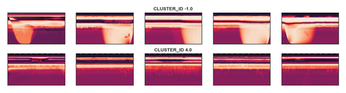Figure 6: Specular images (abnormal and normal bevels) for product X.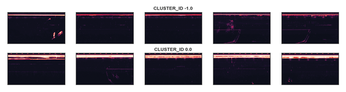Figure 7: Scatter images (abnormal and normal bevels) for product X.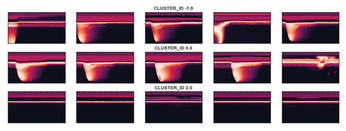Figure 8: Phase images (abnormal and normal bevels) for product X.

Although you can clearly see differences between the image clusters, it is possible that every silicon wafer has the same distribution of cluster IDs, which provide no ability to differentiate between wafers. To investigate this, stacked bar charts were plotted for each product-detector combination, where counts were grouped by wafer, using the command in Listing 17 (see Figure 9).

Listing 17

Cluster ID Stacked Bar Chart

```dfPLUS[(dfPLUS['Detector'] == thisDetector) & (dfPLUS['PROD'] == thisProd)][['Cluster','WAF','INSP_TIME']].\
groupby(['WAF','INSP_TIME','Cluster']).\
size().unstack().\
plot(sort_columns='INSP_TIME',kind='bar', stacked=True)```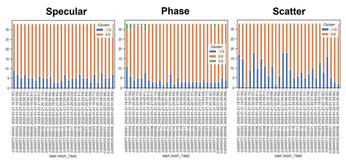Figure 9: Stacked bar charts are plotted by product and image type for product X.

The specular images in Figure 9 show little variation between wafers. The phase images show more interesting behavior with a third cluster ID appearing on a selection of wafers. And perhaps unsurprisingly, the scatter images show an elevated level of scatter.

Differences between products (wafers) can be observed; what remains to be seen is if these differences are useful indicators of yield or reliability. Additionally, the significance of the differences could be determined by using Fisher's exact test . The possibility of individual cluster IDs approaching zero observations makes the application of a Chi-square test  inappropriate in this case.

#### Conclusion

It is certainly possible to segregate abnormal bevel images from those that can be considered normal by means of unsupervised machine learning. This approach is not necessarily appropriate for all image classification applications, but this does not mean that you should overlook it. As always, you should aim to employ the simplest solution where possible. In certain cases, the extraction of texture and form from images can provide a simple solution in conjunction with PCA and DBSCAN.

Unsupervised machine learning bypasses the train, validate, and test cycle. However, the end result in this unsupervised machine learning approach is not a model that can be applied to new images to generate a cluster ID. Instead, the feature extraction, standardization, and PCA generate a set of coordinates that can be compared with the parameter space obtained from the historical data by means of the k-nearest neighbors algorithm . This process provides a cluster ID for each new image.

Infos

1. Smyth, Padhraic, et al. "Knowledge Discovery in Large Image Databases: Dealing with Uncertainties in Ground Truth." In: Advances in Knowledge Discovery and Data Mining (AAAI/MIT Press, Menlo Park, CA, 1995), pp. 109-120
3. pandas: https://pandas.pydata.org/pandas-docs/stable/getting_started/index.html
4. PIL: https://python-pillow.org/
5. cv2: https://pypi.org/project/opencv-python/
6. mahotas: https://pypi.org/project/mahotas/
7. NumPy: https://numpy.org/doc/stable/user/quickstart.html
8. Merging methods: https://pandas.pydata.org/docs/user_guide/merging.html
9. sklearn StandardScaler: https://scikit-learn.org/stable/modules/generated/sklearn.preprocessing.StandardScaler.html
10. sklearn PCA: https://scikit-learn.org/stable/modules/generated/sklearn.decomposition.PCA.html
11. "Indoor Navigation with Machine Learning" by Roland Pleger, Linux Magazine, issue 255, February 2022, https://www.linux-magazine.com/index.php/Issues/2022/255/Machine-Learning
12. sklearn DBSCAN: https://scikit-learn.org/stable/modules/generated/sklearn.cluster.DBSCAN.html
13. Fisher's exact test: https://mathworld.wolfram.com/FishersExactTest.html
14. Chi-square test: https://www.jmp.com/en_be/statistics-knowledge-portal/chi-square-test.html
15. k-nearest neighbor algorithm: https://www.ibm.com/topics/knn

The Author

Garry Tuohy has been surviving in the semiconductor industry for longer than a radiation-hardened processor. He also enjoys astronomy and dreaming of fanciful uses for microcontrollers.

Express-Checkout as PDF
Price \$2.95
(incl. VAT)

SINGLE ISSUES

SUBSCRIPTIONS

## Related content

• Machine Learning

We explore some machine learning techniques with a simple missing person app.

• Machine Learning

"I won't make this mistake again," you promise yourself. In other words, you'll learn from experience. If you translate experience into data, computers can do that, too. We'll introduce you to the fundamental forms of machine learning.

• Treasure Hunt

A geolocation guessing game based on the popular Wordle evaluates a player's guesses based on the distance from and direction to the target location. Mike Schilli turns this concept into a desktop game in Go using the photos from his private collection.

• Perl – k-means Clusters

A human observer can register clusters in a two-dimensional set of points at a glance. Artificial intelligence has a harder time getting it done; however, the relatively simple k-means method delivers usable results.

• Proxmox VE

The Proxmox Virtual Environment has developed from an insider’s tip to a free VMware ESXi/ vSphere clone. We show you how to get started setting up a PVE high-availability cluster.

# Support Our Work

Linux Magazine content is made possible with support from readers like you. Please consider contributing when you've found an article to be beneficial.

## News

• ### PipeWire 1.0 Officially Released

PipeWire was created to take the place of the oft-troubled PulseAudio and has finally reached the 1.0 status as a major update with plenty of improvements and the usual bug fixes.

The latest version of the RHEL alternative is now available and brings back cloud and container images for ppc64le along with plenty of new features and fixes.

• ### Ubuntu Budgie Shifts How to Tackle Wayland

Ubuntu Budgie has yet to make the switch to Wayland but with a change in approaches, they're finally on track to making it happen.

• ### TUXEDO's New Ultraportable Linux Workstation Released

The TUXEDO Pulse 14 blends portability with power, thanks to the AMD Ryzen 7 7840HS CPU.

• ### AlmaLinux Will No Longer Be "Just Another RHEL Clone"

With the release of AlmaLinux 9.3, the distribution will be built entirely from upstream sources.

• ### elementary OS 8 Has a Big Surprise in Store

When elementary OS 8 finally arrives, it will not only be based on Ubuntu 24.04 but it will also default to Wayland for better performance and security.

• ### OpenELA Releases Enterprise Linux Source Code

With Red Hat restricting the source for RHEL, it was only a matter of time before those who depended on that source struck out on their own.

• ### StripedFly Malware Hiding in Plain Sight as a Cryptocurrency Miner

A rather deceptive piece of malware has infected 1 million Windows and Linux hosts since 2017.

• ### Experimental Wayland Support Planned for Linux Mint 21.3

As with most Linux distributions, the migration to Wayland is in full force. While some distributions have already made the move, Linux Mint has been a bit slower to do so.

• ### Window Maker Live 0.96.0-0 Released

If you're a fan of the Window Maker window manager, there's a new official release of the Linux distribution that champions the old-school user interface.